Chapter Notes: Force and Laws of Motion

# Force and Laws of Motion Chapter Notes - Science Class 9

 Table of contentsWhat is Force?Balanced and Unbalanced ForcesNewton’s Laws of Motion1. Newton’s First Law of Motion (Law of Inertia)Inertia and Mass2. Newton’s Second Law of Motion3. Newton’s Third Law of MotionLaw of Conservation of Momentum (Old Syllabus)## What is Force?

A force is an effort that changes the state of an object at rest or at motion. It can change an object’s direction and velocity. Force can also change the shape of an object.

It is the force that enables us to do any work.

To do anything, either we pull or push the object. Therefore, pull or push is called force.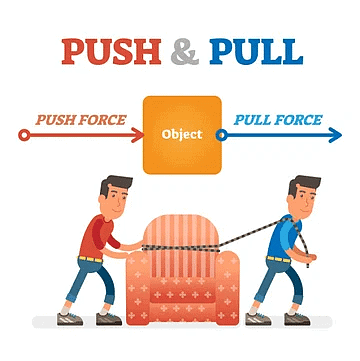Push or Pull is called ForceExample: To open a door, either we push or pull it. A drawer is pulled to open and pushed to close.

### Effects of Force

• Force can make a stationary body move.
Example: A football can be set to move by kicking it, i.e. by applying a force.
• Force can stop a moving body.
Example: By applying brakes, a running cycle or a running vehicle can be stopped.
• Force can change the direction of a moving object.
Example: By applying force, i.e. by moving the handle, the direction of a running bicycle can be changed. Similarly, by moving steering, the direction of a running vehicle is changed.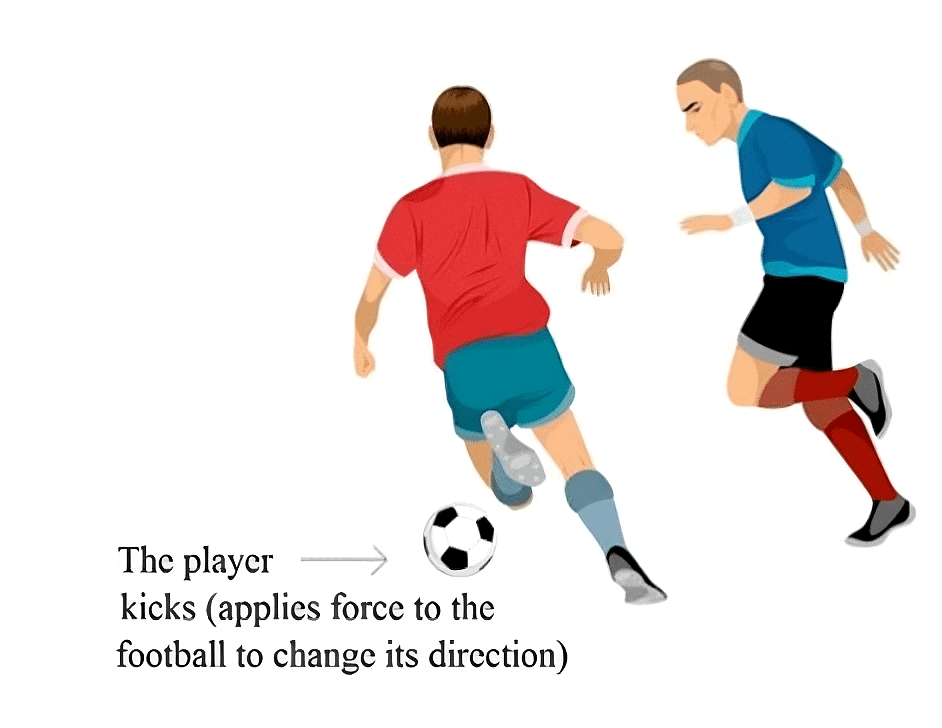Effects of Force

• Force can change the speed of a moving body.
Example: By accelerating, the speed of a running vehicle can be increased, or by applying brakes the speed of a running vehicle can be decreased.
• Force can change the shape and size of an object.
Example: By hammering, a block of metal can be turned into a thin sheet. By hammering, a stone can be broken into pieces.

Question for Chapter Notes: Force and Laws of Motion
Try yourself:Which of the following statements best defines force?

## Balanced and Unbalanced Forces

In physics, forces can be classified as balanced or unbalanced based on their effects on an object's motion. Mentioned below are the details of both these forces: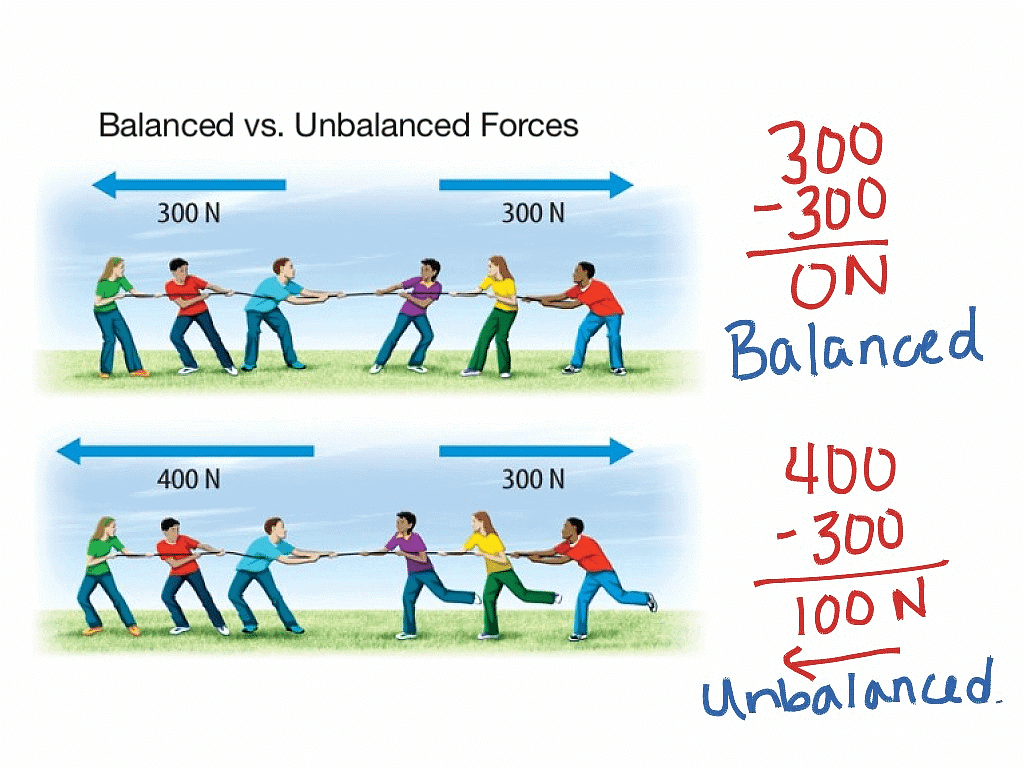Balanced and Unbalanced Forces

### Balanced Forces

If the resultant of applied forces is equal to zero, it is called balanced forces.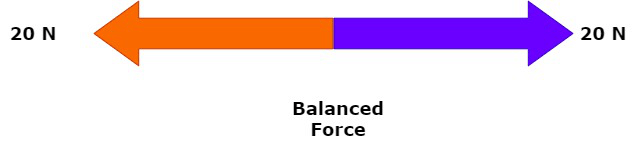Balanced Force

• Example: In the tug of war if both the team apply similar magnitude of forces in opposite directions, the rope does not move in either side. This happens because of balanced forces in which the resultant of applied forces become zero.
• Balanced forces do not cause any change of state of an object. Balanced forces are equal in magnitude and opposite in direction.
• Balanced forces can change the shape and size of an object.
Example: When forces are applied from both sides over a balloon, the size and shape of the balloon are changed.

### Unbalanced Forces

If the resultant of applied forces are greater than zero, the forces are called unbalanced forces. An object at rest can be moved because of applying unbalanced forces.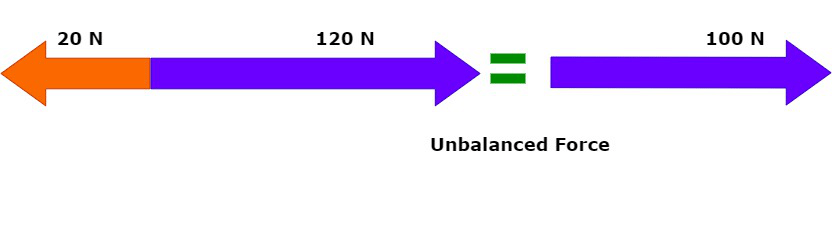Unbalanced Force

Unbalanced forces can do the following:

• Move a stationary object.
• Increase the speed of a moving object.
• Decrease the speed of a moving object.
• Stop a moving object.
• Change the shape and size of an object.

Question for Chapter Notes: Force and Laws of Motion
Try yourself:Which of the following statements best describes balanced forces?

### Galileo's Idea of Motion

Galileo, first of all, said that objects move at a constant speed when no forces act on them.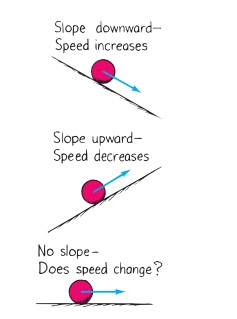Galileo's Idea of Motion

• This means if an object is moving on a frictionless path and no other force is acting upon it, the object would be moving forever. That is, there is no unbalanced force working on the object.
• But practically it is not possible for any object. Because to attain the condition of zero, the unbalanced force is impossible.
• Force of friction, Force of air, and many other forces are always acting upon an object.

## Newton’s Laws of Motion

Newton studied the ideas of Galileo and gave the three laws of motion. These laws are known as Newton’s laws of motion:
(i) Newton’s First Law of Motion (Law of Inertia).
(ii) Newton’s Second Law of Motion.
(iii) Newton’s Third Law of Motion.

## 1. Newton’s First Law of Motion (Law of Inertia)

Any object remains in the state of rest or uniform motion along a straight line until it is compelled to change the state by applying an external force.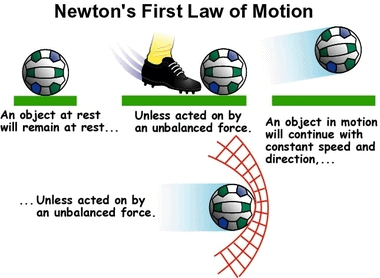Illustration of Newton's First Law of Motion

Explanation

• If any object is in the state of rest, then it will remain in rest until an external force is applied to change its state.
• Similarly, an object will remain in motion until an external force is applied over it to change its state.
• This means all objects resist changing their state. The state of any object can be changed by applying external forces only.

Examples of Newton’s First Law of Motion in Everyday Life

• A person standing inside a bus falls backwards when the bus starts moving suddenly.
Explanation: This happens because the person and bus both are at rest while the bus is not moving, but as the bus starts moving, the legs of the person start moving along with the bus, but the rest portion of his body has the tendency to remain in rest. Because of this, the person falls backwards; if he is not alert.
• A person standing inside a moving bus falls forward if the driver applies brakes suddenly.
Explanation: This happens because when the bus is moving, the person standing in it is also in motion along with the bus. But when the driver applies brakes the speed of the bus decreases suddenly or the bus comes into the state of rest suddenly, in this condition the legs of the person, which are in contact with the bus come in rest while the rest part of his body tends to remain in motion. Because of this person falls forward if he is not alert.
• Before hanging the wet clothes over the laundry line, usually many jerks are given to the clothes to get them dried quickly. Because of jerks, droplets of water from the pores of the cloth fall on the ground, and a reduced amount of water in clothes dries them quickly.
Explanation: This happens because when suddenly clothes are made in motion by giving jerks, the water droplets in them have the tendency to remain in rest and they are separated from clothes and fall on the ground.
• When the pile of coin on the carom-board is hit by a striker, the coin only at the bottom moves away leaving the rest of the pile of the coin in the same place.
Explanation: This happens because when the pile is struck with a striker, the coin at the bottom comes in motion while the rest of the coin in the pile has the tendency to remain in the rest and they vertically fall on the carom-board and remain at the same place.

## Inertia and Mass

• The property of an object because of which it resists getting disturbed its state is called inertia.
In other words, the natural tendency of an object that resists the change in the state of motion or rest of the object is called inertia.
• The inertia of an object is measured by its mass. Inertia is directly proportional to the mass of the object.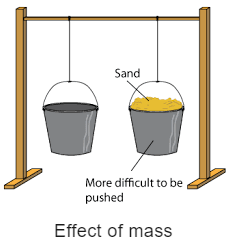Inertia increases with an increase in mass and decreases with a decrease in mass.

• A heavy object will have more inertia than the lighter one.
Since a heavy object has more inertia, thus it is difficult to push or pull a heavy box over the ground than the lighter one.

## 2. Newton’s Second Law of Motion

The rate of change of momentum of an object is proportional to the applied unbalanced force in the direction of the force.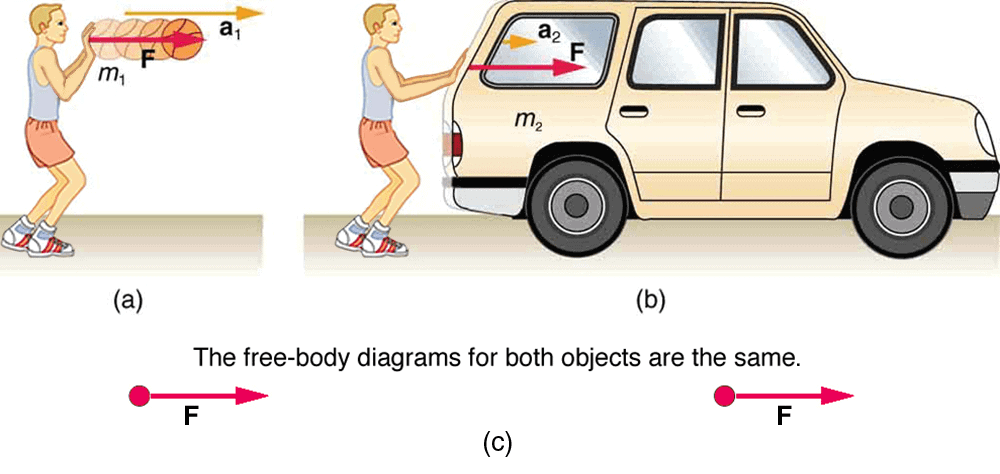Illustration of Force depending on Mass and Acceleration.

(a) Momentum

• Momentum is the power of motion of an object.
• The product of velocity and mass is called momentum.
Momentum is denoted by ‘p’.
• Therefore, Momentum of the object = Mass × Velocity (p = m × v),
where p = momentum, m = mass of the object and v = velocity of the object.

Some explanations to understand the momentum:

• A person gets injured in the case of hitting a moving object, such as stone, pebbles, or anything because of the momentum of the object.
• Even a small bullet can kill a person when it is fired from a gun because of its momentum due to great velocity.
• A person gets injured severely when hit by a moving vehicle because of the momentum of the vehicle due to mass and velocity.
(b) Momentum and Mass
• Since momentum is the product of mass and velocity (p = m × v) of an object. This means momentum is directly proportional to mass and velocity. Momentum increases with the increase of either mass or velocity of an object.
• This means if a lighter and a heavier object is moving with the same velocity, then the heavier object will have more momentum than the lighter one.
• If a small object is moving with great velocity, it has tremendous momentum. And because of momentum, it can harm an object more severely.
Example: A small bullet having a little mass even kills a person when it is fired from a gun.
• Usually, road accidents prove more fatal because of high speed than slower speed. This happens because vehicles running at high speed have greater momentum compared to a vehicle running with a slower speed.

(c) Unit of Momentum

• We know that,
Momentum (p) = m × v
• SI unit of mass = kg
• SI unit of velocity = m/s

Therefore,

p = kg × m/s ⇒ p = kgm/s

The momentum of an object which is in the state of rest:
Let, an object with mass ‘m’ is in the rest.
Since, the object is at rest, therefore, its velocity, v = 0
∵ Momentum = mass × velocity
⇒ p = m × 0 = 0
Thus, the momentum of an object in the rest, i.e. non-moving is equal to zero.

### Numerical Problems Based on Momentum

Example 1: What will be the momentum of a stone that has a mass of 10 kg when it is thrown with a velocity of 2 m/s?
Solution:
Mass (m) = 10 kg, Velocity (v) = 2 m/s.
∵ Momentum (p) = Mass (m) × Velocity (v)
p = 10 kg × 2 m/s = 20 kg m/s.
Thus, the momentum of the stone = 20 kg m/s.

Example 2: Calculate the momentum of a bullet of 25 g when it is fired from a gun with a velocity of 100 m/s.
Solution:
Given, Velocity of the bullet (v) = 100 m/s,
Mass of the bullet (m) = 25 g = 25/1000 kg = 0.025 kg.
∵ p = m × v
p = 0.025 × 100 = 2.5 kg m/s.
Thus, Momentum of the bullet  = 2.5 kg m/s.

Example 3: Calculate the momentum of a bullet having a mass of 25 g is thrown using a hand with a velocity of 0.1 m/s.
Solution:
Given, Velocity of the bullet (v) = 0.1 m/s,
Mass of the bullet (m) = 25 g = 25/1000 kg = 0.025 kg.
∵ Momentum (p) = Mass (m) × Velocity (v)
p = 0.025 kg × 0.1 = 0.0025 kg m/s
Thus, Momentum of the bullet = 0.0025 kg m/s.

Example 4: The mass of a goods lorry is 4000 kg and the mass of goods loaded on it is 20000 kg. If the lorry is moving with a velocity of 2 m/s, what will be its momentum?
Solution: Velocity (v) = 2 m/s
Mass of lorry = 4000 kg
Mass of goods on the lorry = 20000 kg.
Total mass (m) on the lorry = 4000 kg + 20000 kg = 24000 kg
∵ Momentum (p) = Mass (m) × Velocity (v)
p = 24000 kg × 2 = 48000 kg m/s
Thus, Momentum of the lorry = 48000 kg m/s.

Example 5: A car having a mass of 1000 kg is moving with a velocity of 0.5 m/s. What will be its momentum?
Solution: Velocity of the car (v) = 0.5 m/s
Mass of the car (m) = 1000 kg.
∵ Momentum (p) = Mass (m) × Velocity (v)
p = 1000 kg × 0.5 m/s = 500 kg m/s
Thus, Momentum of the car = 500 kg m/s.

(d) Mathematical Expression of Second Law of Motion
Suppose,
Mass of an object = m kg
Initial velocity of an object = u m/s,
Final velocity of an object = v m/s.
Initial momentum, p1 = mu, Final momentum, p2 = mv.
Change in momentum = Final momentum – Initial momentum
Change in momentum = mv – mu = m(v – u)
Rate of change of momentum = Change in momentum/Time taken
Rate of change of momentum = m(v-u)/t
According to 2nd law, this rate of change is momentum is directly proportional to force, i.e.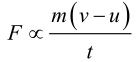Newton's Second Law of Motion

We know that:
a = (v-u)/t (From 1st equation of motion)
⇒ F = kma, where k is a constant.
Its value can be assumed as 1.
F = 1 × m × a = ma
SI unit = kgms-2 or Newton
• 1 Newton: When an acceleration of 1 m/s2 is seen in a body of mass 1 kg, then the force applied on the body is said to be 1 Newton.

(e) Proof of Newton’s First Law of Motion from the Second Law
The first law states that if external force F = 0, then a moving body keeps moving with the same velocity, or a body at rest continues to be at rest.
F = 0
We know, F = m(v-u)/t

• A body is moving with initial velocity u then,
m(v-u)/t = 0 ⇒ v – u = 0
⇒ v = u
Thus, final velocity is also same.
•  A body is at rest i.e., u = 0
⇒ u = v = 0
Hence, the body will continue to be at rest.

## 3. Newton’s Third Law of Motion

According to Newton's third Law of Motion, for every action, there is an equal and opposite reaction.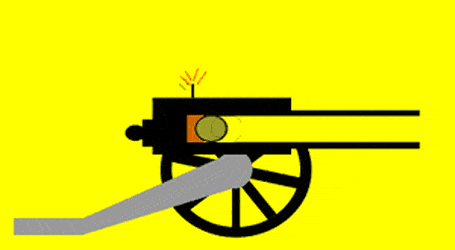Illustration of 3rd Law of Motion.

Applications

• Walking is enabled by the 3rd law.
• A boat moves back when we deboard it.
• A gun recoils.
• Rowing of a boat.

## Law of Conservation of Momentum (Old Syllabus)

When two (or more) bodies act upon one another, their total momentum remains constant (or conserved) provided no external forces are acting.
Initial momentum = Final momentum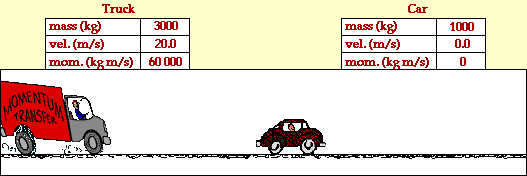Law of Conservation of Momentum
• Suppose, two objects A and B each of mass m1 and mass m2 are moving initially with velocities u1 and u2, strike each other after time t and start moving with velocities v1 and v2 respectively.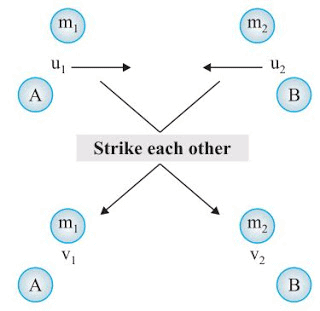Illustration of the Law of Conservation of Momentum

• Now,
Initial momentum of object A = m1u1, Initial momentum of object B = m2u2
Final momentum of object A = m1v1,Final momentum of object B = m2v2
• So,
Rate of change of momentum in A, F1 = (m1v1 - m1u1)t = m1(v1 - u1)/t ....(i)
Rate of change of momentum in B, F2 = (m2v2 - m2u2)t =  m2(v2 - u2)/t....(ii)
• We know from the 3rd law of motion, F1 = − F2
• So, m1(v1 - u1)/t = -m2(v2 - u2)/t
⇒ m1v1 – m2u2 = −m2v2 + m1u1
⇒ m1u1 + m2u2 = m1v1 + m2v2
• Thus, Initial momentum = Final momentum

### Numerical Problems Based on Conservation of Momentum

Example 1: A bullet of mass 20 g is fired horizontally with a velocity of 150 m/s from a pistol of mass 2 kg. Find the recoil velocity of the pistol.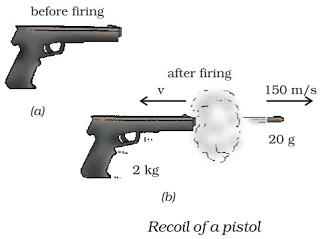Solution:
Given:
Mass (m1) of bullet = 20 g = 0.02 kg
Mass (m2) of pistol = 2 kg
v1 for bullet = 150
Initially, the bullet is inside the gun and it is not moving.
∵ Mass = m1+m2 = (0.02 + 2) kg = 2.02 kg, and u1 = 0
⇒ Initial momentum = 2.02 × 0 = 0  ....(i)
Let the velocity of the pistol be v2.
Final momentum = m1v1 + m2v= 0.02×150 + 2v2 ...(ii)
∵ Initial momentum = Final momentum
(0.02×150)/100 + 2v2 = 0   [From equations (i) and (ii)]
⇒ 3 + 2v2 = 0
⇒ 2v2 = −3
⇒ v2 = −1.5 m/s.
(−) ve sign indicates that gun recoils in direction opposite to that of the bullet.

Example 2: Two hockey players viz A of mass 50 kg is moving with a velocity of 4 m/s and another one B belonging to the opposite team with mass 60 kg is moving with 3 m/s, get entangled while chasing and fall. Find the velocity with which they fall down and in which direction?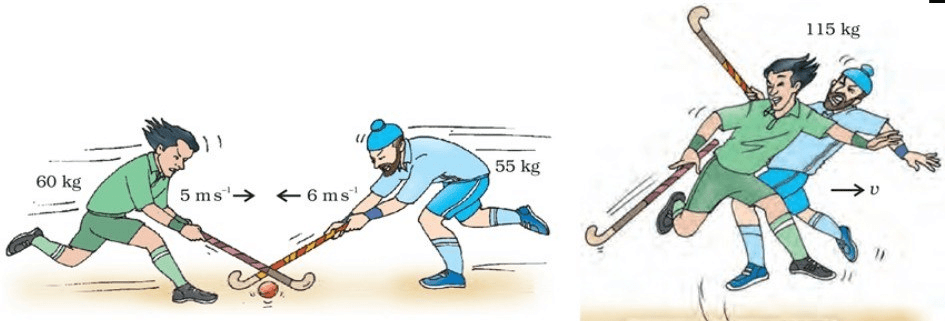A Collision of Two Hockey Players: (a) Before the Collision and (b) After the Collision

Solution:
Given, mA = 50 kg, uA = 4 m/s, mB = 60 kg, uB = 3 m/s.
Initial momentum A = mAuA = 50 × 4 = 200 kgm/s,
Initial momentum B = mBuB = 60 × 3 = 180 kgm/s.
Total initial momentum = 200 + 180 = 380 kgm/s ....(i)
⇒ Final momentum = (mA + mB)v = (50 + 60)v = 110v  ....(ii)
According to the law of conservation of momentum:
Initial momentum = Final momentum
⇒ 380  = 110v
⇒ v = 380/110 = 3.454 m/s.
The document Force and Laws of Motion Chapter Notes | Science Class 9 is a part of the Class 9 Course Science Class 9.
All you need of Class 9 at this link: Class 9

## Science Class 9

66 videos|352 docs|97 tests

## FAQs on Force and Laws of Motion Chapter Notes - Science Class 9

 1. What is force?Ans. Force is a push or pull that is applied on an object to change its state of motion or shape. It is a vector quantity and is measured in Newtons (N).
 2. What are the effects of force?Ans. The effects of force can be classified as the change in the state of motion and change in shape. When a force is applied on an object, it can change the object's velocity, direction, or both. It can also cause deformation or change in the shape of the object.
 3. What are the three laws of motion proposed by Newton?Ans. Newton's three laws of motion are: 1. Law of Inertia: An object at rest will remain at rest, and an object in motion will remain in motion at a constant velocity unless acted upon by an external force. 2. Law of Acceleration: The acceleration of an object is directly proportional to the net force acting on it and inversely proportional to its mass. 3. Law of Action and Reaction: For every action, there is an equal and opposite reaction.
 4. How do Newton's laws of motion explain the motion of objects?Ans. Newton's laws of motion explain the motion of objects by providing a mathematical framework that describes how objects move under the influence of external forces. The laws explain how objects remain in their state of motion or change their motion in response to applied forces.
 5. What is the relationship between force and acceleration?Ans. The relationship between force and acceleration is described by Newton's second law of motion. According to this law, the acceleration of an object is directly proportional to the net force acting on it and inversely proportional to its mass. Thus, a larger force results in a larger acceleration, while a larger mass results in a smaller acceleration for the same force.

## Science Class 9

66 videos|352 docs|97 testsExplore Courses for Class 9 examSignup to see your scores go up within 7 days! Learn & Practice with 1000+ FREE Notes, Videos & Tests.
10M+ students study on EduRev
Track your progress, build streaks, highlight & save important lessons and more!
Related Searches

,

,

,

,

,

,

,

,

,

,

,

,

,

,

,

,

,

,

,

,

,

;